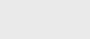3年前 (2020-10-14) |   抢沙发  576

• 阶乘函数（通常表示为n！）是一个经典的数学具有递归定义的自然函数。
• 英国尺子具有递归模式，这是分形的一个简单示例结构。
• 二进制搜索是最重要的计算机算法之一。它允许以高效地定位我们需要的数十亿个数据集条目的数量。
• 计算机的文件系统具有递归结构，其中目录可以任意深入嵌套在其他目录中。递归算法被广泛用于探索和管理这些文件系统。

## 递归示例

### 阶乘函数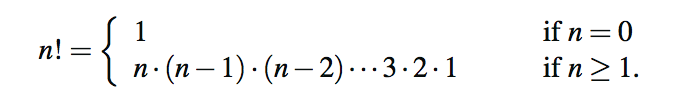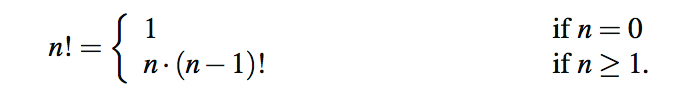``````public static int factorial(int n) throws IllegalArgumentException {
if (n < 0)
throw new IllegalArgumentException( ); // argument must be nonnegative
else if (n == 0)
return 1; // base case
else
return n ∗ factorial(n−1); // recursive case
}``````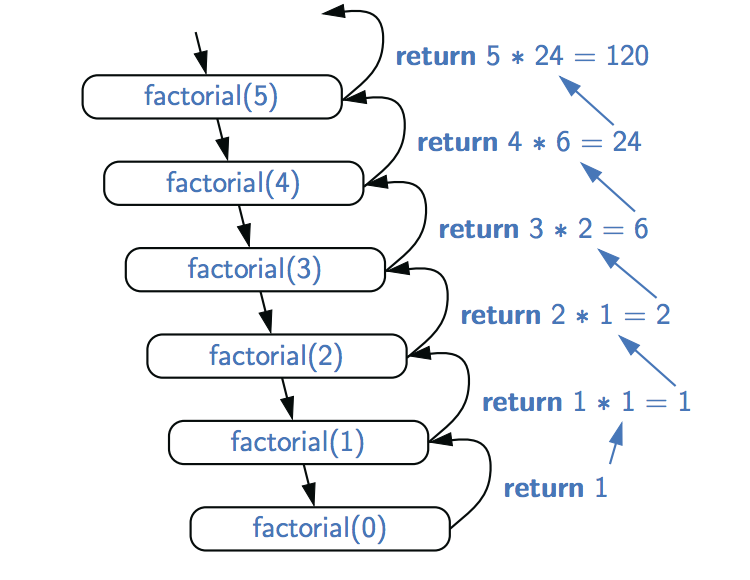### 画英国尺子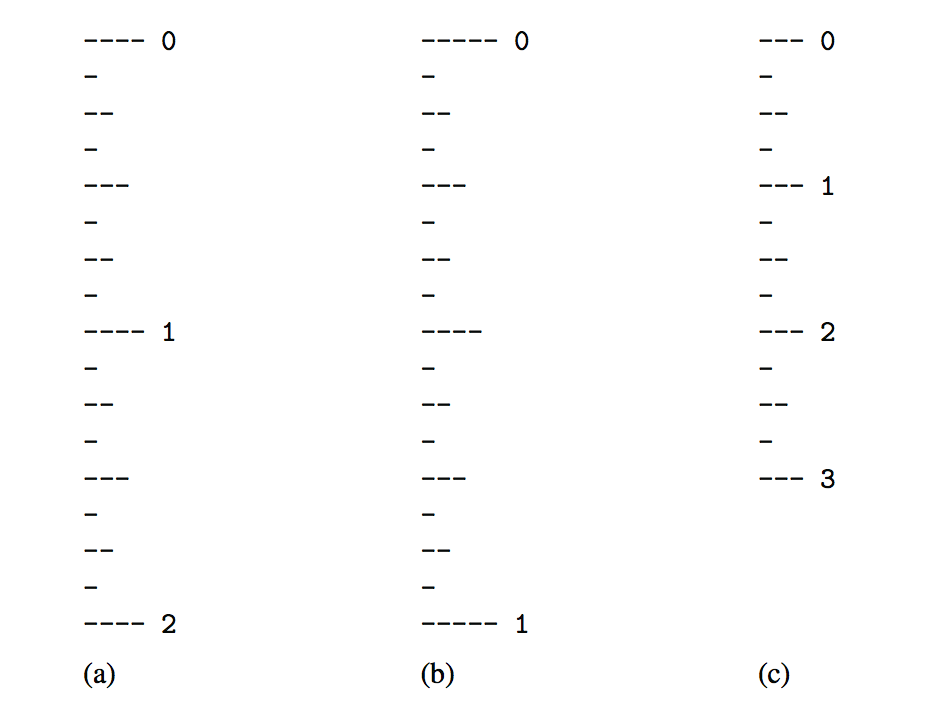### 递归作图法

• 中心刻度长度L−1的间隔
• 长度为L的单个刻度
• 中心刻度长度L−1的间隔

``````/∗∗ Draws an English ruler for the given number of inches and major tick length. ∗/
public static void drawRuler(int nInches, int majorLength) {
drawLine(majorLength, 0); // draw inch 0 line and label
for (int j = 1; j <= nInches; j++) {
drawInterval(majorLength − 1); // draw interior ticks for inch
drawLine(majorLength, j); // draw inch j line and label
}
}
private static void drawInterval(int centralLength) {
if (centralLength >= 1) { // otherwise, do nothing
drawInterval(centralLength − 1); // recursively draw top interval
drawLine(centralLength); // draw center tick line (without label)
drawInterval(centralLength − 1); // recursively draw bottom interval
}
}
private static void drawLine(int tickLength, int tickLabel) {
for (int j = 0; j < tickLength; j++)
System.out.print("-");
if (tickLabel >= 0)
System.out.print(" " + tickLabel);
System.out.print("\n");
}
/∗∗ Draws a line with the given tick length (but no label). ∗/
private static void drawLine(int tickLength) {
drawLine(tickLength, −1);
}``````### 注册